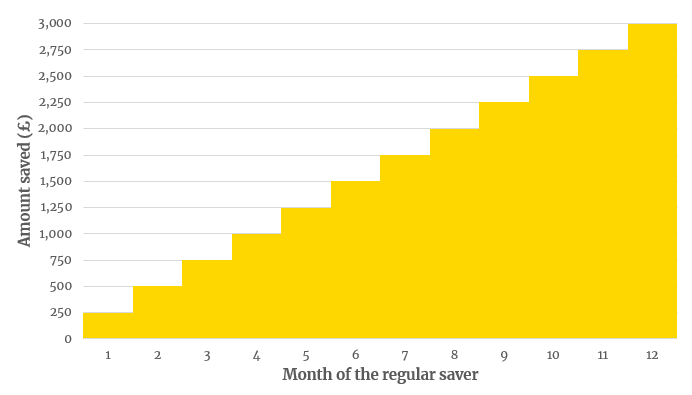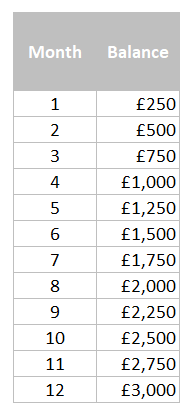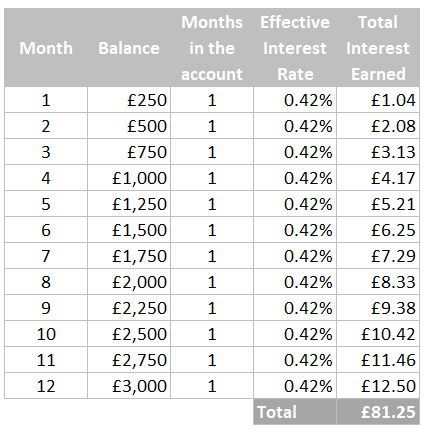# How you can calculate the interest on a regular saver

## What is a regular saver?

Regular savers are a savings product which require you to add some money into the account every month. Interest is normally paid at the term, which is normally 1 year.

For example, if you open a 1-year regular saver and deposit £250 a month, in the end you will you will receive back your £3,000 (£250 x 12) with the interest.

## Why am I writing about how to calculate the interest?

I opened my first regular saver a while ago because I was excited by the headline 5% interest rate, which was way the 1.5% in my current account. As in the example above, I deposited £250 into my regular saver for 12 months.

At the end of the year I was expecting 5% x £3000 = £150 back in interest. However, I received £80.64. This was initially baffling for me so I investigated the calculations in more depth.

This post aims to explain the maths a simple way. Before starting let’s discuss the advantages / disadvantages of a regular saver.

## Advantages of a Regular Saver?

1. The top account’s interest rate is likely to be way above what’s being offered in your current account, but as this blog post will explain, the maths on how much interest you receive is a bit more than multiplying two numbers together.

2. It is structured to help you save towards a big goal by saving a bit at a time. Want to put away a but of money every month to go on a big holiday costing £2,400 next year? Locking away just £200 a month into your regular saver will help you get there before you know it.

1. Any money deposited into your account is locked away until the end of the account (normally a year). Withdrawing at any point before then will most likely result in a loss of interest.

2. Some accounts require you to save the same amount every month, which you have to define when opening the account. This can get tricky if your circumstances change in a few months’ time.

## How can you calculate the interest?

Note this method doesn’t take into compounding so the amount you’ll end up receiving will be slightly more. My aim here isn’t to be mathematically accurate, but to provide an intuitive way to get very close to the actual number.

### Step 1: Monthly interest

Before jumping into how to calculate the amount you’d receive at the end of a regular saver, I would like to take a step back and discuss how you’d calculate the interest earned on £250 if you had it in the bank for 1 month at 5%.

As you only had it in the bank for 1 month, you would divide the annual interest rate by 12 to get the monthly interest rate:

Monthly interest rate = 5%/12

You then times this by the amount you had in the bank to get the interest earned: 5%/12 * £250 = £1.04

If instead you had this £250 in the bank for 8 months, you would take the annual interest rate, divide it by 12 and the multiply by 8 to get the interest rate you’d earn over 8 months.

Hence, the total interest rate you would earn = 5%/12*8 * £250 = £8.33

### Step 2: The amount of money in your regular saver over time

Your regular saver normally starts with you depositing a certain amount of money into your account, let’s say £250 a month as in my initial example.

You then add another £250 a at the beginning of the next 11 months, to end with a closing balance of £3000 in 12 months’ time.### Step 3: Calculating the interest

Based on this, it now makes a bit more sense why my simple calculation of 5% * £3,000 didn’t work before: £3,000 was not in this account for the whole year. In fact, I only had £3,000 in the account for 1 month. I had £250 in the account for 1 month, £500 for 1 month and so on...

Therefore, to calculate the end interest amount, you would need to split up your account balance as per the amount of time you had the money in the bank as per this table.In this case, for each of these months your effective interest rate would be 5%/12 = 0.42%.

You can then calculate for each month how much interest you would have earnt in each of those periods by multiplying the amount in the bank by the effective interest rate of 0.42%.Adding up the total in interest earned column we get to a total of £81.25. This ties out very closely to the £81.44 that I recently ended up receiving at the of my 5% regular saver with M&S.

I agree it’s not a perfect match but I wanted to keep the maths simple and intuitive so everyone could understand.

### I just want a quick calculation!

For a quick calculation, you can use half the advertised interest rate times the end amount in interest.

Comparing to our pervious example, this would calculate as £3,000 * 2.5% = £75, which is close but of course not as good as the previous method.

### What are the top accounts at the moment?

As of 26th January 2019, there are a few 5% Regular Savers:

• First Direct (have to switch to have switched to their 1st account)

• M&S Bank (have to have switched to them)

• Nationwide (Current account holders)

• HSBC (Advance customers only otherwise 3%)

### Conclusion

I hope this blog post cleared up some of the mathematics behind the interest. If you have any questions, as always, feel free to contact us at hello@onepounddd.com